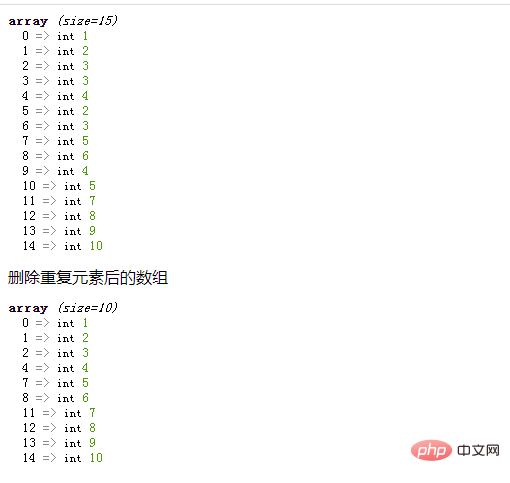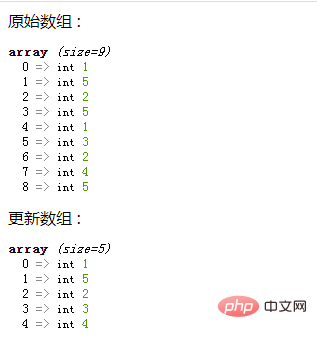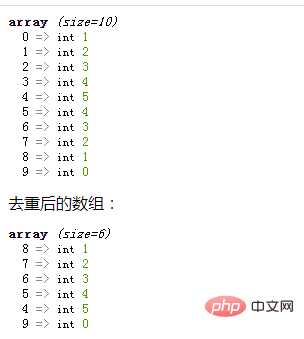# php怎么删除数组重复元素php中提供多种方法来删除数组重复元素。下面我们来了解一下。

array_unique() 函数用于移除数组中重复的值。如果两个或更多个数组值相同，只保留第一个值，其他的值被移除。

```<?php
\$array = array(1,2,3,3,4,2,3,5,6,4,5,7,8,9,10);
var_dump(\$array);

\$result = array_unique(\$array);
echo "删除重复元素后的数组";
//var_dump(array_values(\$filtered_array));
var_dump(\$result);
?>```array_flip() 函数用于反转/交换数组中的键名和对应关联的键值。

```<?php
\$a = array(1, 5, 2, 5, 1, 3, 2, 4, 5);
// 输出原始数组
echo "原始数组 :";
var_dump(\$a);
// 通过使用翻转键和值移除重复值
\$a = array_flip(\$a);
// 通过再次翻转键和值来恢复数组元素
\$a = array_flip(\$a);
// 重新排序数组键
\$a = array_values(\$a);
// 输出更新后的数组
echo "更新数组 :";
var_dump(\$a);
?>``````\$res1=[];
\$res2=[];```

```foreach (\$array as \$k1 => \$v1){  //在每次循环中会将当前数组的值赋给 \$v1，键名赋给 \$k1
\$res1[\$v1]=\$k1;
}```

```foreach (\$res1 as \$k2 => \$v2){
\$res2[\$v2]=\$k2;
}```

```<?php
function f(\$arr){
var_dump(\$arr);
\$res1=[];
\$res2=[];
foreach(\$arr as \$k1=>\$v1){
\$res1[\$v1]=\$k1;
}
foreach (\$res1 as \$k2 => \$v2){
\$res2[\$v2]=\$k2;
}
echo "去重后的数组：";
var_dump(\$res2);
}

\$arr=array(1,2,3,4,5,4,3,2,1,0);
f(\$arr);
?>```#### 相关文章推荐

• php数组下标怎么求和• php数组怎么把key（键）转为值• php数组怎么去除0值• php数组中怎么进行并集求和• php数组指针什么用• php数组是传值还是引用
1/1